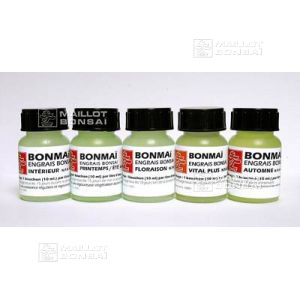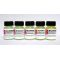The Japanese Bonsai specialist
Direct order Contact Help / Services NewsletterBonsai fertilisers 5 bottle gift pack

› Bonsai soil and fertiliser › Fertilizerref. : 5246

12,00

Available quantity : 21Order

Description

Five 60ml bottles of liquid fertilizer for bonsai. 1 for indoor bonsai, 1 for flowers, 1 Vital Plus for all bonsai, 1 to use in spring/summer and 1 for autumn. The ideal gift for a bonsai enthusiast, this five-pack of mini bottles will provide indoor and outdoor bonsai with the perfect conditions to grow all year round.

Dosage: 10 ml or 1 capful of fertiliser per 1 litre of fresh water. Water onto wet earth.

#bonsai 7.6 #fertilizer 4 #pack 3.4 #gift 3.4 #fertilisers 3.1 #bottles 2.7 #indoor 2.6 #bottle 2.6 #water 2.5 #five 2.4

Formule
(( ROUND((CHAR_LENGTH(b.article_nom)-CHAR_LENGTH(REPLACE(b.article_nom, 'bonsai', '')))/LENGTH('bonsai')) + ROUND((CHAR_LENGTH(b.article_description)-CHAR_LENGTH(REPLACE(b.article_description, 'bonsai', '')))/LENGTH('bonsai')) ) * 7.6) + (( ROUND((CHAR_LENGTH(b.article_nom)-CHAR_LENGTH(REPLACE(b.article_nom, 'pack', '')))/LENGTH('pack')) + ROUND((CHAR_LENGTH(b.article_description)-CHAR_LENGTH(REPLACE(b.article_description, 'pack', '')))/LENGTH('pack')) ) * 3.4) + (( ROUND((CHAR_LENGTH(b.article_nom)-CHAR_LENGTH(REPLACE(b.article_nom, 'gift', '')))/LENGTH('gift')) + ROUND((CHAR_LENGTH(b.article_description)-CHAR_LENGTH(REPLACE(b.article_description, 'gift', '')))/LENGTH('gift')) ) * 3.4) + (( ROUND((CHAR_LENGTH(b.article_nom)-CHAR_LENGTH(REPLACE(b.article_nom, 'fertilisers', '')))/LENGTH('fertilisers')) + ROUND((CHAR_LENGTH(b.article_description)-CHAR_LENGTH(REPLACE(b.article_description, 'fertilisers', '')))/LENGTH('fertilisers')) ) * 3.1) + (( ROUND((CHAR_LENGTH(b.article_nom)-CHAR_LENGTH(REPLACE(b.article_nom, 'bottles', '')))/LENGTH('bottles')) + ROUND((CHAR_LENGTH(b.article_description)-CHAR_LENGTH(REPLACE(b.article_description, 'bottles', '')))/LENGTH('bottles')) ) * 2.7) + (( ROUND((CHAR_LENGTH(b.article_nom)-CHAR_LENGTH(REPLACE(b.article_nom, 'indoor', '')))/LENGTH('indoor')) + ROUND((CHAR_LENGTH(b.article_description)-CHAR_LENGTH(REPLACE(b.article_description, 'indoor', '')))/LENGTH('indoor')) ) * 2.6) + (( ROUND((CHAR_LENGTH(b.article_nom)-CHAR_LENGTH(REPLACE(b.article_nom, 'bottle', '')))/LENGTH('bottle')) + ROUND((CHAR_LENGTH(b.article_description)-CHAR_LENGTH(REPLACE(b.article_description, 'bottle', '')))/LENGTH('bottle')) ) * 2.6) + (( ROUND((CHAR_LENGTH(b.article_nom)-CHAR_LENGTH(REPLACE(b.article_nom, 'water', '')))/LENGTH('water')) + ROUND((CHAR_LENGTH(b.article_description)-CHAR_LENGTH(REPLACE(b.article_description, 'water', '')))/LENGTH('water')) ) * 2.5) + (( ROUND((CHAR_LENGTH(b.article_nom)-CHAR_LENGTH(REPLACE(b.article_nom, 'five', '')))/LENGTH('five')) + ROUND((CHAR_LENGTH(b.article_description)-CHAR_LENGTH(REPLACE(b.article_description, 'five', '')))/LENGTH('five')) ) * 2.4) + (( ROUND((CHAR_LENGTH(b.article_nom)-CHAR_LENGTH(REPLACE(b.article_nom, 'enthusiast', '')))/LENGTH('enthusiast')) + ROUND((CHAR_LENGTH(b.article_description)-CHAR_LENGTH(REPLACE(b.article_description, 'enthusiast', '')))/LENGTH('enthusiast')) ) * 2)

Secure paymentDelivery

Our logistic partners :04 74 55 23 48
Pépinière MAILLOT-BONSAÏ
Le Bois Frazy
01990 RELEVANT - FRANCE
on appointment